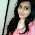Figuring out budgets and or income levels from prices - FreeEconHelp.com, Learning Economics... Solved!

## 12/16/11

This post will focus more on algebra, but it will use algebra to answer an economics question.  This type of question asks you to find out an individual’s income, if they give you two different scenarios and a price for one of the goods.  The trick to answering problems like this is to set up the problem correctly.  Once you have set up the problem, answering is a simple exercise with algebra.

If Hellen spends all her income on Lemons & Oranges Hellen can just afford 30 Lemons & 8 Oranges per day.   She could also use her entire budget to buy 6 Lemon & 14 Oranges per day. The price of Lemon is \$6.How much is Hellen's income per day?

This problem is the classic case of two equations, two unknowns (meaning unknown variables).  Here the two equations are the different combinations of goods that Hellen is able to purchase.  The two unknowns are the price of oranges and the total income allocated to Hellen each day.

First let’s set up the first scenario, where Hellen is able to purchase 30 lemons and 8 oranges.  If we denote the price of oranges as X, and the total income received per day as Y, we will get the following equation:

30 lemons * \$6 (the price of lemons) + 8 oranges * \$X (the price of oranges) = \$Y (total income received per day

Or

30*6 + 8*X = Y

The second scenario has Hellen purchasing 6 lemons and 14 oranges.  This equation would look like:

6*6 + 14*X = Y

Luckily, both of these equations are already solved for Y (income) and we know that Y = Y so we can set the first equation equal to the second equation, which gives us:

30*6 + 8*X = 6*6 + 14*X

Or

180 + 8X = 36 + 14X

Now we can subtract 36 from both sides, and subtract 8 from both sides to get:

144 = 6X

Now we divide both sides by 6 to get X = 24

This gives us the price of oranges which we can use to determine what the daily income amount is.  So plugging the price of oranges into the first two equations gives us:

180+8*24 = 180+192 = 372

And

36+14*24 = 36+336 = 372

So the Hellen’s daily income given the above information is \$372.

1.2.